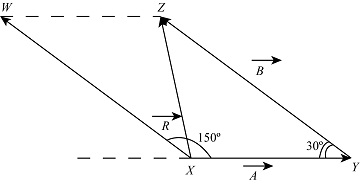# Hiker Harry walks 3 km east then 4 km 30 degrees north of west. A. How far (in km) is Harry from...

## Question:

Hiker Harry walks {eq}\rm 3 \ km {/eq} east then {eq}\rm 4 \ km \ 30 \ degrees {/eq} north of west.

A. How far {eq}\rm (in \ km) {/eq} is Harry from the starting point?

B. Measured from the east {eq}( \rm +x-axis) {/eq}, what is the direction of Harry's position?

The Vector addition of two vectors not done by regular scalar operation, so they are done by the triangle law of the parallelogram law of the vector addition to getting the resultant of the two vectors.

Given data

• The distance traveled by Harry in east direction is: {eq}\overrightarrow A = 3\;{\rm{km}} {/eq}
• The distance traveled by Harry in north of west direction is: {eq}\overrightarrow B = 4\;{\rm{km}} {/eq}

The below figure represents the total distance traveled by the Harry.In vectors the distance is always measure tail to tail, the angle between {eq}\overrightarrow A {/eq} and {eq}\overrightarrow B is 150^\circ {/eq}.

The expression for the resultant of two vectors is,

{eq}R = \sqrt {{A^2} + {B^2} + 2AB\cos \theta } {/eq}

Substitute the given values in the above expression.

{eq}\begin{align*} R &= \sqrt {{{\left( {3\;{\rm{km}}} \right)}^2} + {{\left( {4\;{\rm{km}}} \right)}^2} + 2\left( {3\;{\rm{km}}} \right)\left( {4\;{\rm{km}}} \right)\cos 150^\circ } \\ &= 2.053\;{\rm{km}} \end{align*} {/eq}

Thus, the distance of the Harry from the starting point is {eq}2.053\;{\rm{km}}. {/eq}

b)

Referred to the above figure Harry direction at the final point is North-west.

Thus, the direction at the end point is in north-west direction.